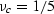# A differential scheme for elastic properties of rocks with dry or saturated cracks

James G. Berryman,Steven R. Pride,and Herbert F. Wang(1)
x M A C D E F G K L P R S T V W Int Bdy

# ABSTRACT

Differential effective medium (DEM) theory is applied to the problem of estimating physical properties of elastic media with penny-shaped cracks, filled either with air or liquid. These cracks are assumed to be randomly oriented. It is known that such a model captures many of the essential physical features of fluid-saturated or partially saturated rocks. By making the assumption that the changes in certain factors depending only on Poisson's ratio do not strongly affect the results, it is possible to decouple the equations for bulk (K) and shear (G) modulus, and then integrate them analytically. The validity of this assumption is then tested by integrating the full DEM equations numerically. The analytical and numerical curves for both K and G are in very good agreement over the whole porosity range of interest. Justification of the Poisson's ratio approximation is also provided directly by the theory, which shows that, as porosity tends to 100%, Poisson's ratio tends towards small positive values for dry, cracked porous media and tends to one-half for liquid saturated samples. A rigorous stable fixed point is obtained for Poisson's ratio,, of dry porous media, where the location of this fixed point depends only on the shape of the voids being added. Fixed points occur atfor spheres, andfor cracks,being the aspect ratio of penny-shaped cracks. Results for the elastic constants are then compared and contrasted with results predicted by Gassmann's equations and with results of Mavko and Jizba, for both granite-like and sandstone-like examples. Gassmann's equations do not predict the observed liquid dependence of the shear modulus G at all. Mavko and Jizba predict the observed dependence of shear modulus on liquid bulk modulus for small crack porosity, but fail to predict the observed behavior at higher porosities. In contrast, the analytical approximations derived here give very satisfactory agreement in all cases for both K and G.